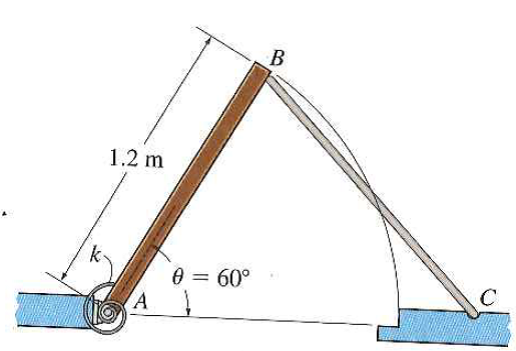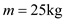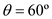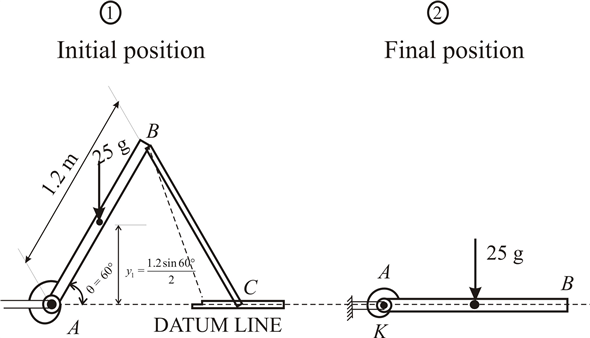Problem

# The uniform rectangular door panel has a mass of 25 kg and is held in equilibrium above...

The uniform rectangular door panel has a mass of 25 kg and is held in equilibrium above the horizontal at the position θ = 60° by rod BC. Determine the required stiffness of the torsional spring at A, so that the door's angular velocity becomes zero when the door reaches the closed position (θ = 0°) once the supporting rod BC is removed. The spring is undeformed when θ = 60°.#### Step-by-Step Solution

Solution 1

Given data:

Mass of uniform rectangular doorInitial position,Let required torsional stiffness of spring be k

The diagram in initial and final positions is as shown below:$$y_{1}=\frac{1.2 \sin 60^{\circ}}{2}$$

$$=0.5196 \mathrm{~m}$$

$$\theta_{e}=60^{\circ}=\pi / 3$$ radians

POTENTIAL ENERGY:-

Initial position:

The gravitational potential energy of door panel

$$\left(V_{\text {door }}\right)_{1}=+W y_{1}$$

$$=+25 \times 9.81 \times 0.5196$$

$$=+127.4319 \mathrm{~J}$$

As springs are un stretched in initial position

So, potential energy becomes

$$V_{1}=\left(V_{\text {door }}\right)_{1}+\left(V_{\text {spring }}\right)_{1}$$

$$=127.4319+0$$

$$=127.43 \mathrm{~J}$$

Final position:

As door panel $$\mathrm{AB}$$ has center of gravity on datum line, So it has zero gravitational potential energy.

The spring is stretched by angle $$=60^{\circ}$$ in final position

So elastic potential energy of torsional spring,

$$\left(V_{\text {spring }}\right)_{2}=\frac{1}{2} k \theta_{e}^{2}$$

$$=\frac{1}{2} \times k \times\left(\frac{60^{\circ}}{180} \times \pi\right)^{2}$$

$$=\frac{1}{2} k\left(\frac{\pi}{3}\right)^{2}$$

Therefore the final potential energy is

$$V_{2}=\left(V_{\text {door }}\right)_{2}+\left(V_{\text {spring }}\right)_{2}$$

$$=0+\frac{1}{2} \times k(\pi / 3)^{2}$$

$$=\frac{\pi^{2}}{18} k$$

KINETIC ENERGY:

As system is at rest initially and also angular velocity of door reaches zero in final position. So in initial and final kinetic energy $$=0$$

$$T_{1}=0$$

$$T_{2}=0$$

Using conservation of energy principle,

$$V_{1}+T_{1}=V_{2}+T_{2}$$

$$127.43+0=\frac{\pi^{2}}{18} k+0$$

$$k=\frac{18 \times 127.43}{\pi^{2}}$$

$$k=232.4 \mathrm{~N} \cdot \mathrm{m} / \mathrm{rad}$$

Torsional stiffness of spring, $$k=232.4 \mathrm{~N} \cdot \mathrm{m} / \mathrm{rad}$$